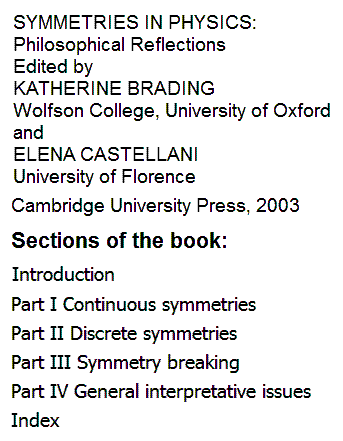# Log24

## Saturday, February 18, 2012

### Symmetry

Filed under: General,Geometry — m759 @ 7:35 PM

From the current Wikipedia article "Symmetry (physics)"—

"In physics, symmetry includes all features of a physical system that exhibit the property of symmetry—that is, under certain transformations, aspects of these systems are 'unchanged', according to a particular observation. A symmetry of a physical system is a physical or mathematical feature of the system (observed or intrinsic) that is 'preserved' under some change.

A family of particular transformations may be continuous  (such as rotation of a circle) or discrete  (e.g., reflection of a bilaterally symmetric figure, or rotation of a regular polygon). Continuous and discrete transformations give rise to corresponding types of symmetries. Continuous symmetries can be described by Lie groups while discrete symmetries are described by finite groups (see Symmetry group)."….

"A discrete symmetry is a symmetry that describes non-continuous changes in a system. For example, a square possesses discrete rotational symmetry, as only rotations by multiples of right angles will preserve the square's original appearance."

Note the confusion here between continuous (or discontinuous) transformations  and "continuous" (or "discontinuous," i.e. "discrete") groups .

This confusion may impede efforts to think clearly about some pure mathematics related to current physics— in particular, about the geometry of spaces made up of individual units ("points") that are not joined together in a continuous manifold.

For an attempt to forestall such confusion, see Noncontinuous Groups.

For related material, see Erlanger and Galois as well as the opening paragraphs of Diamond Theory

Symmetry is often described as invariance under a group of transformations. An unspoken assumption about symmetry in Euclidean 3-space is that the transformations involved are continuous.

Diamond theory rejects this assumption, and in so doing reveals that Euclidean symmetry may itself  be invariant under rather interesting groups of non-continuous (and a-symmetric) transformations. (These might be called noncontinuous  groups, as opposed to so-called discontinuous  (or discrete ) symmetry groups. See Weyl's Symmetry .)

For example, the affine group A on the 4-space over the 2-element field has a natural noncontinuous and asymmetric but symmetry-preserving action on the elements of a 4×4 array. (Details)

(Version first archived on March 27, 2002)

Update of Sunday, February 19, 2012—

The abuse of language by the anonymous authors
of the above Wikipedia article occurs also in more
reputable sources. For instance—Some transformations referred to by Brading and Castellani
and their editees as "discrete symmetries" are, in fact, as
linear transformations of continuous spaces, themselves
continuous  transformations.

This unfortunate abuse of language is at least made explicit
in a 2003 text, Mathematical Perspectives on Theoretical
Physics
(Nirmala Prakash, Imperial College Press)—

"… associated[*] with any given symmetry there always exists
a continuous or a discrete group of transformations….
A symmetry whose associated group is continuous (discrete)
is called a continuous  (discrete ) symmetry ." — Pp. 235, 236